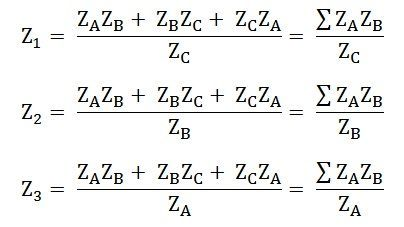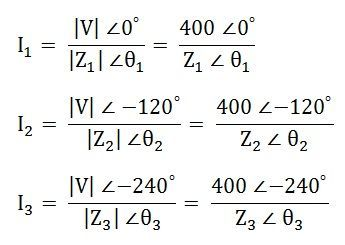# Star to Delta and Delta to Star Conversion

The conversion or transformation or replacement of the star connected load network to a Delta connected network and similarly, a delta connected network to a star network is done by Star to Delta or Delta to Star Conversion.

Contents:

## Star to Delta ConversionIn star to delta conversion, the star-connected load is to be converted into delta connection. Suppose we have a star connected load as shown in the figure above, and it has to be converted into a delta connection as shown in figure B.

The following Delta values are as follows:Hence, if the values of ZA, ZB and ZC are known, therefore by knowing these values and by putting them in the above equations, you can convert a star connection into a delta connection.

## Delta to Star Conversion

Similarly, a delta connection network is given as shown above, in figure B and it has to be transformed into a star connection, as shown above, in the figure A. The following formulas given below are used for the conversion:If the values of Z1, Z2 and Z3 are given, then by putting these values of the Impedances in the above equations, the conversion of delta connection into star connection can be performed.

As Impedance (Z) is the vector quantity, therefore all the calculations are done in Polar and Rectangular form.

### Steps for Analysis of 3 phase unbalanced Delta Connected Load

Let us take an example of Delta connected unbalanced load connected to 400 V, 3 phase supply as shown in the figure below:The following steps are given below to solve the 3 phase unbalanced delta connected loads.

Step 1 – Solve, each phase current I1, I2 and I3 as in the single-phase circuit.

AsStep 2 – Compute the line currents IL1, IL2 and IL3, in rectangular form.Step 3 – Now, compute the power of different phases.Step 4 – Calculate the total power by the equation shown below.A similar procedure will be followed for solving the unbalanced Star connected load. Firstly, the star impedance will be converted into the corresponding delta impedance. The remaining calculations will be followed as for the Delta connected load as shown above.